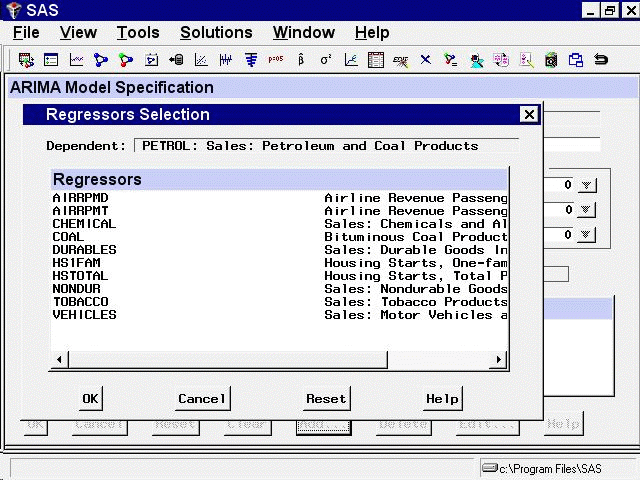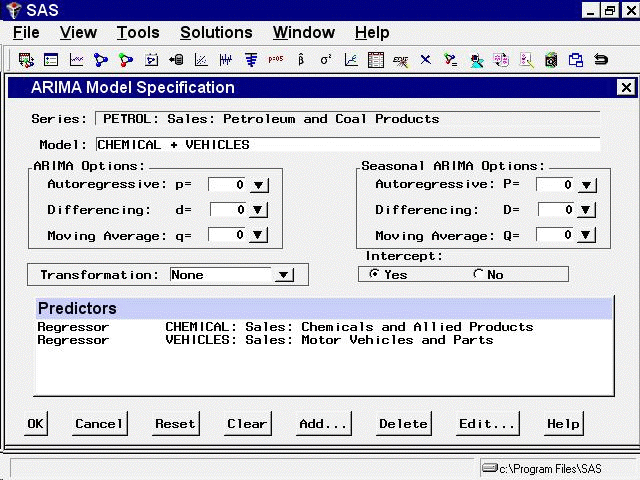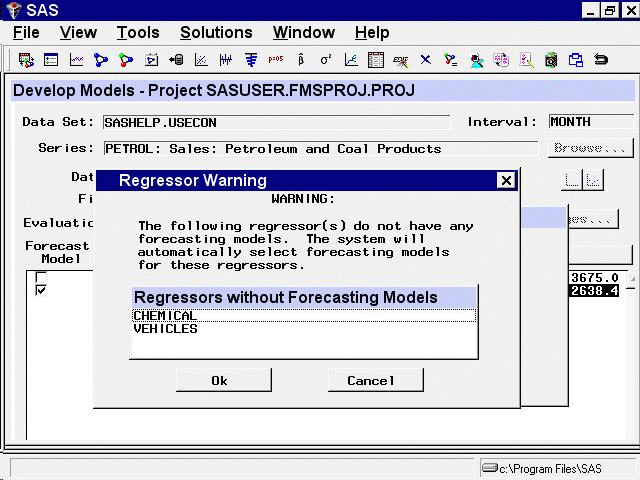# Using Predictor Variables

## Regressors

From the Develop Models window, select `Fit ARIMA Model.` From the ARIMA Model Specification window, select `Add` and then select `Regressors` from the menu (shown in Figure 50.1). This displays the `Regressors Selection` window, as shown in Figure 50.8. This window allows you to select any number of other series in the input data set as regressors to predict the dependent series.

Figure 50.8: Regressors Selection WindowFor this example, select `CHEMICAL, Sales: Chemicals and Allied Products`, and `VEHICLES, Sales: Motor Vehicles and Parts`. (Note: You do not need to use the CTRL key when selecting more than one regressor.) Then select the `OK` button. The two variables you selected are added to the Predictors list as regressor type predictors, as shown in Figure 50.9.

Figure 50.9: Regressors SelectedYou must have forecasts of the future values of the regressor variables in order to use them as predictors. To do this, you can specify a forecasting model for each regressor, have the system automatically select forecasting models for the regressors, or supply predicted future values for the regressors in the input data set.

Even if you have supplied future values for a regressor variable, the system requires a forecasting model for the regressor. Future values that you supply in the input data set take precedence over predicted values from the regressor’s forecasting model when the system computes the forecasts for the dependent series.

Select the `OK` button. The system starts to fit the regression model but then stops and displays a warning that the regressors that you selected do not have forecasting models, as shown in Figure 50.10.

Figure 50.10: Regressors Needing Models WarningIf you want the system to create forecasting models automatically for the regressor variables by using the automatic model selection process, select the `OK` button. If not, you can select the `Cancel` button to abort fitting the regression model.

For this example, select the `OK` button. The system now performs the automatic model selection process for CHEMICAL and VEHICLES. The selected forecasting models for CHEMICAL and VEHICLES are added to the model lists for those series. If you switch the current time series in the Develop Models window to CHEMICAL or VEHICLES, you will see the model that the system selected for that series.

Once forecasting models have been fit for all regressors, the system proceeds to fit the regression model for PETROL. The fitted regression model is added to the model list displayed in the Develop Models window.